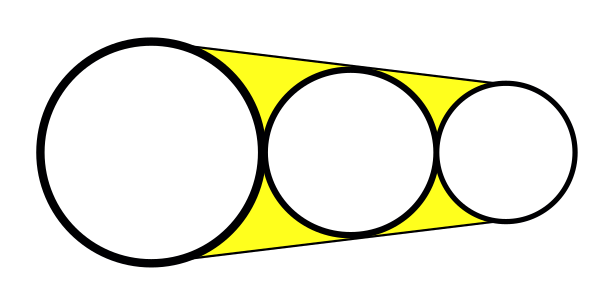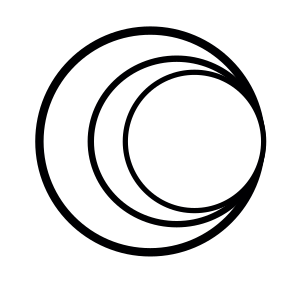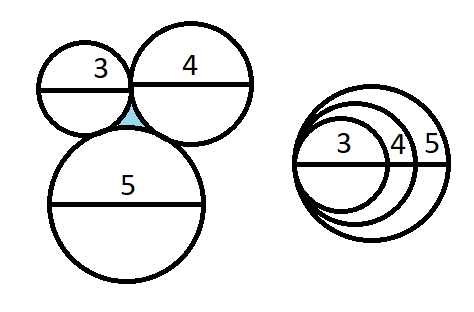# Math mini-contest problem for Day 1 on D.Buzz for April 2021 😎

in D.Buzz2 months ago

Math mini-contest problem for Day 1 on D.Buzz for April 2021 😎

Fang drew 3 circles whose diameters are 3, 4, and 5 cm, respectively, and the circles intersect each other exactly once. What is the area of the region surrounded by the circles but outside the circles?

Sort:

I guess you mean something like the yellow area here:But I'm lazy today, so I chose a different arrangement of the 3 circles:In this case my answer is 0: there is no surrounding area outside the circle

Ha! Nice. I thought it might be the circles in a triangle arrangement so they all touch each other. I like your solution better.

Ha, you're right, a triangle arrangement was probably meant, each circle touching each other once. The task is to find the inside area not covered by the circles. I'll stick with my shortcut for today, feel free to give the proper solution a try :D

# 0 🎯

There is also another answer depending on how you interpreted the word problem (see solution).

## SolutionThere are two ways to draw the circles which "intersect each other exactly once" (as shown on the image). It is either the circles do not overlap each other, or the circles completely overlap one another.

1. In the first scenario, the area of the region inscribed by the three circles is equal to the area of the triangle formed by the combination of the radii of the three circles minus the area of the sector of the three circles. I would like to leave the solution to the reader using both geometry and trigonometry. 😅
2. In the second scenario, since the two smaller circles are inside the larger circle, there is no region that is enclosed by the circles. Therefore, the area of the region is 0 square centimeters. (The unit does not matter here, because the quantity is exactly zero.) 🎯

# Winner: @minus-pi 🏅

1 HIVE has been sent to @minus-pi's Hive account. 💰

• @jfang003's answer was "no answer", but there can be multiple answers to the problem based on how it is interpreted. 😁
• @eturnerx's understanding of the problem is valid, but he did not put an answer. 😮

Mentions: @holovision, @ahmadmanga (@ahmadmangazap), @paultactico2, @appukuttan66, and @dkmathstats 🤓
Special mentions: @chrisrice, @jancharlest, and @mehmetfix 🤯

My guess is "No Solution".

I have no idea about how the circles are placed on the paper so the area surrounded but outside can't be calculated.